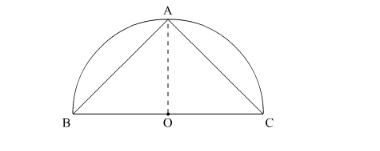# The area of the largest triangle that

Question:

The area of the largest triangle that can be inscribed in a semi-circle of radius r is

(a) $2 r$

(b) $r^{2}$

(c) $r$

(d) $\sqrt{r}$

Solution:

The triangle with the largest area will be symmetrical as shown in the figure.Let the radius of the circle be r.

Hence,

$\operatorname{ar}(\triangle \mathrm{ABC})=\frac{1}{2}(r)(2 r)$

$=r^{2}$ sq. unit